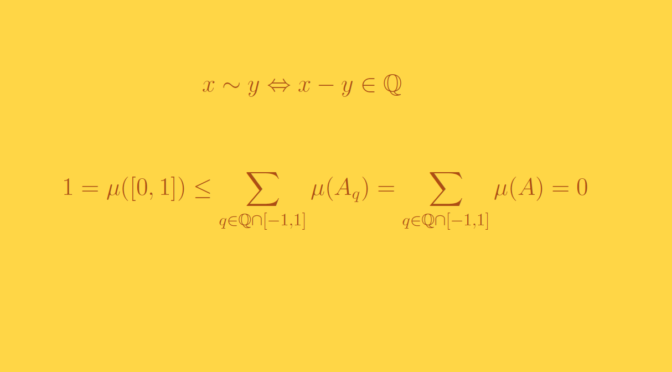# A non-measurable set

We describe here a non-measurable subset of the segment $$I=[0,1] \subset \mathbb R$$.

Let’s define on $$I$$ an equivalence relation by $$x \sim y$$ if and only if $$x-y \in \mathbb Q$$. The equivalence relation $$\sim$$ induces equivalence classes on $$I$$. For $$x \in I$$, it’s equivalence class $$[x]$$ is $$[x] = \{y \in I \ : \ y-x \in \mathbb Q\}$$. By the Axiom of Choice, we can form a set $$A$$ by selecting a single point from each equivalence class.

We claim that the set $$A$$ is not Lebesgue measurable.

For all $$q \in \mathbb Q$$ we denote $$A_q = \{q+x \ : x \in A\}$$. Let’s take $$p,q \in \mathbb Q$$. If it exists $$z \in A_p \cap A_q$$, it means that there exist $$u,v \in A$$ such that
$z= p+u=q+v$ hence $$u-v=q-p=0$$ as $$u,v$$ are supposed to be unique representatives of the classes of the equivalence relation $$\sim$$. Finally if $$p,q$$ are distincts, $$A_p \cap A_q = \emptyset$$.

As Lebesgue measure $$\mu$$ is translation invariant, we have for $$q \in \mathbb Q \cap [0,1]$$ : $$\mu(A) = \mu(A_q)$$ and also $$A_q \subset [0,2]$$. Hence if we denote
$B = \bigcup_{q \in \mathbb Q \cap [0,1]} A_q$ we have $$B \subset [0,2]$$. If we suppose that $$A$$ is measurable, we get
$\mu(B) = \sum_{q \in \mathbb Q \cap [0,1]} \mu(A_q) = \sum_{q \in \mathbb Q \cap [0,1]} \mu(A) \le 2$ by countable additivity of Lebesgue measure (the set $$\mathbb Q \cap [0,1]$$ being countable infinite). This implies $$\mu(A) = 0$$.

Let’s prove now that
$[0,1] \subset \bigcup_{q \in \mathbb Q \cap [-1,1]} A_q$ For $$z \in [0,1]$$, there exists $$u \in A$$ such that $$z \in [u]$$. As $$A \subset [0,1]$$, we have $$q = z-u \in \mathbb Q$$ and $$-1 \le q \le 1$$. And $$z=q+u$$ means that $$z \in A_q$$. This proves the inclusion. However the inclusion implies the contradiction
$1 = \mu([0,1]) \le \sum_{q \in \mathbb Q \cap [-1,1]} \mu(A_q) = \sum_{q \in \mathbb Q \cap [-1,1]} \mu(A) =0$

Finally $$A$$ is not Lebesgue measurable.